# RD Sharma Solutions for Class 9 Maths Chapter 7 Introduction to Euclid's Geometry

## RD Sharma Solutions for Class 9 Maths Chapter 7 – Free PDF Download

RD Sharma Solutions for Class 9 Maths Chapter 7 Introduction To Euclid’s Geometry are provided here to help the students practice and learn the concepts of the Chapter more effectively. Euclid’s Geometry is a fundamental concept that forms the basis for much more advanced topics. Therefore,  the best guide to assist students to understand this concept in-depth is RD Sharma Solutions for Class 9 Maths Chapter 7. Students can download RD Sharma Solutions Class 9  formulated by knowledgeable teachers with the aim to help students secure high marks in exams.

The RD Sharma Solutions are solved in a detailed manner for a better understanding of the concepts, which helps students prepare for their exams at ease. Our expert tutors at BYJU’S have made it possible to help students crack difficult problems. Class 9 is considered amongst the most important class in a student’s life as the topics studied in this class forms the base for higher grades as well. RD Sharma is one of the best textbooks for Mathematics which is designed in accordance to get complete knowledge about the subject.

## Download PDF of RD Sharma Solutions for Class 9 Maths Chapter 7 Introduction to Euclid’s Geometry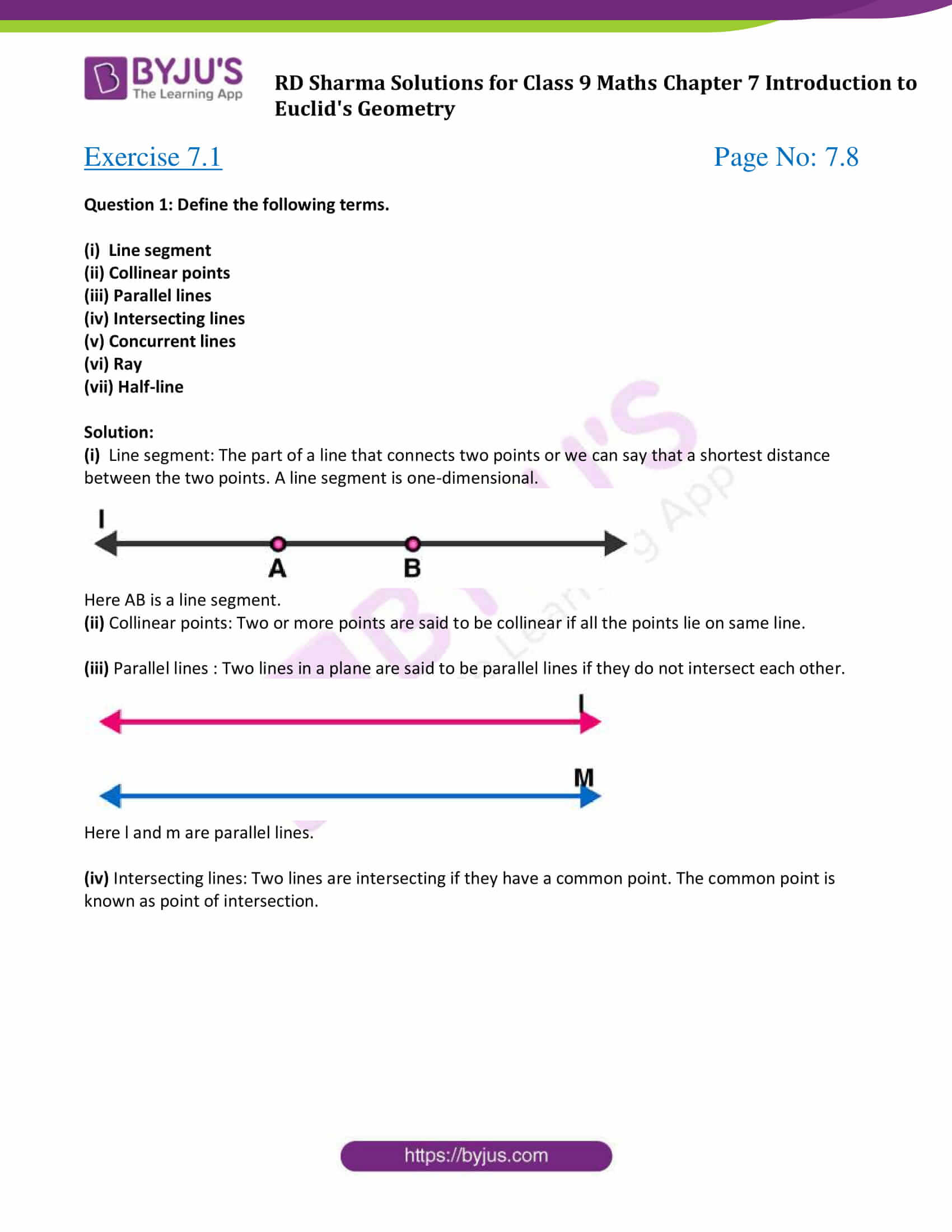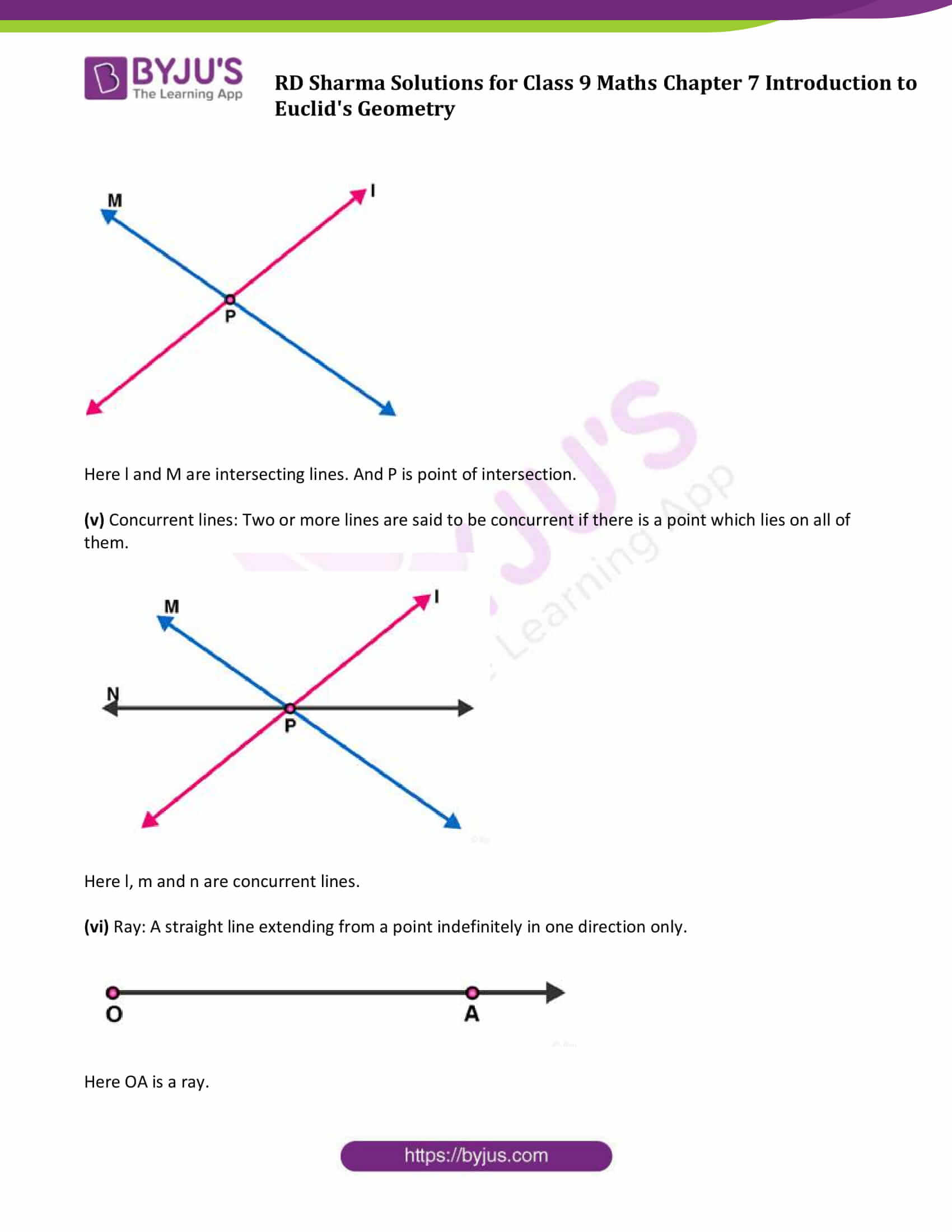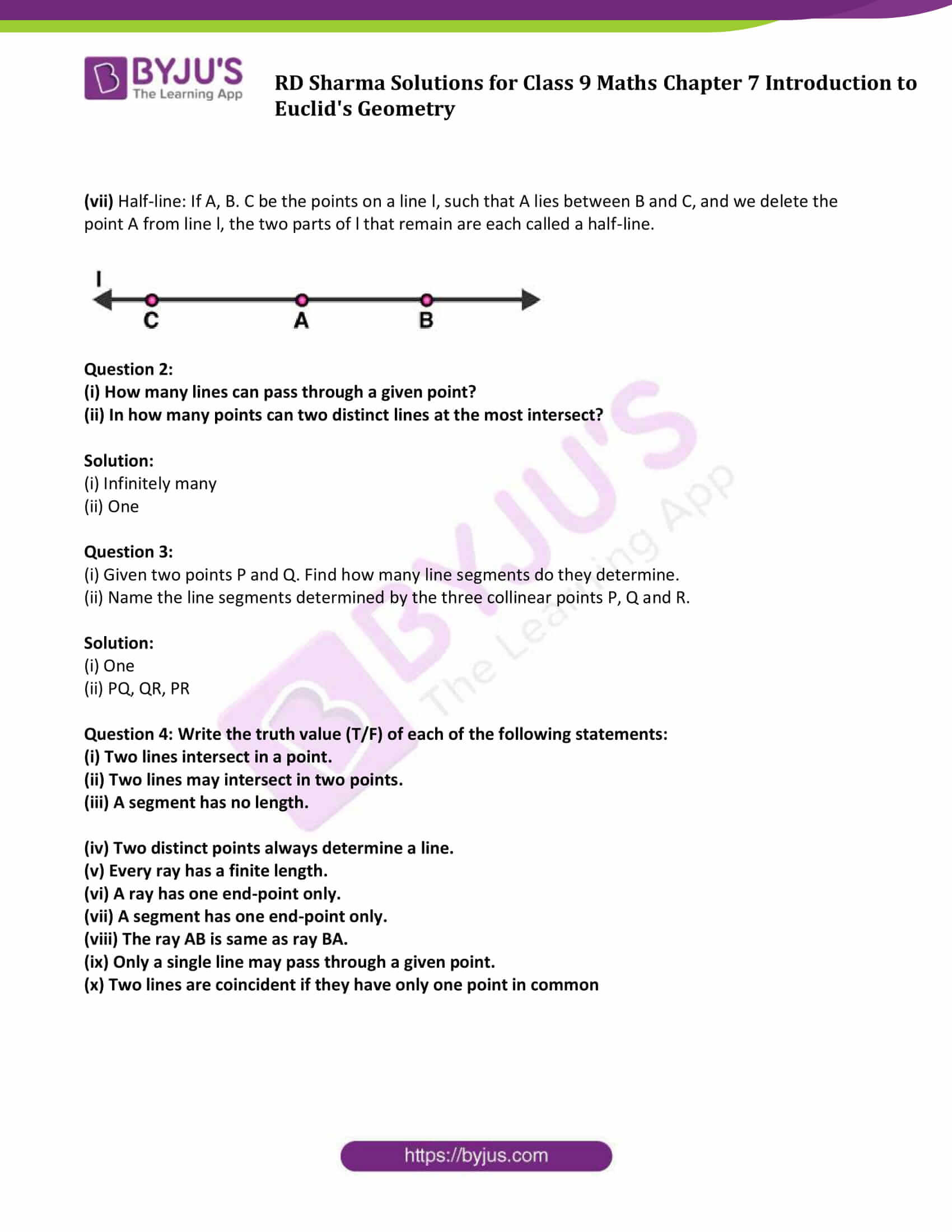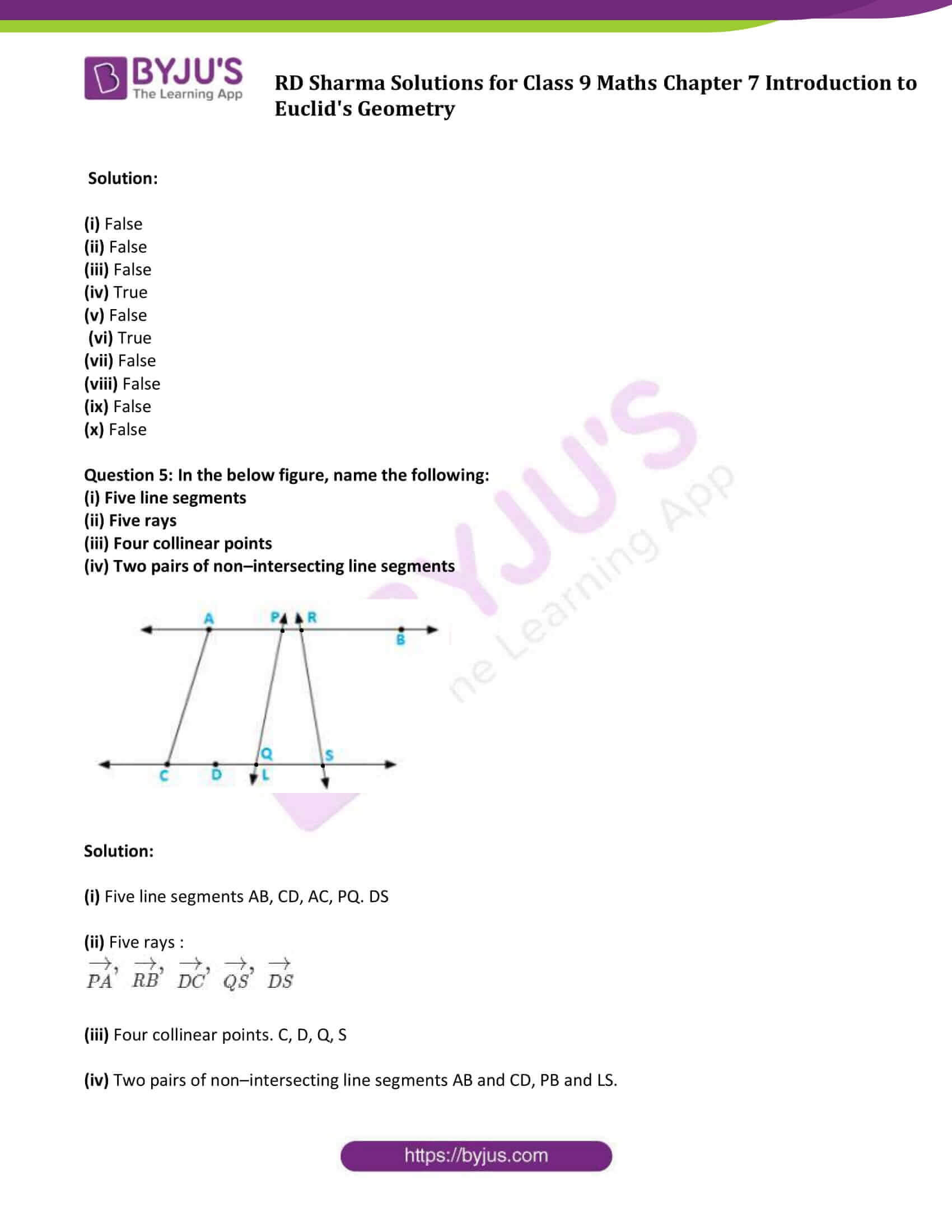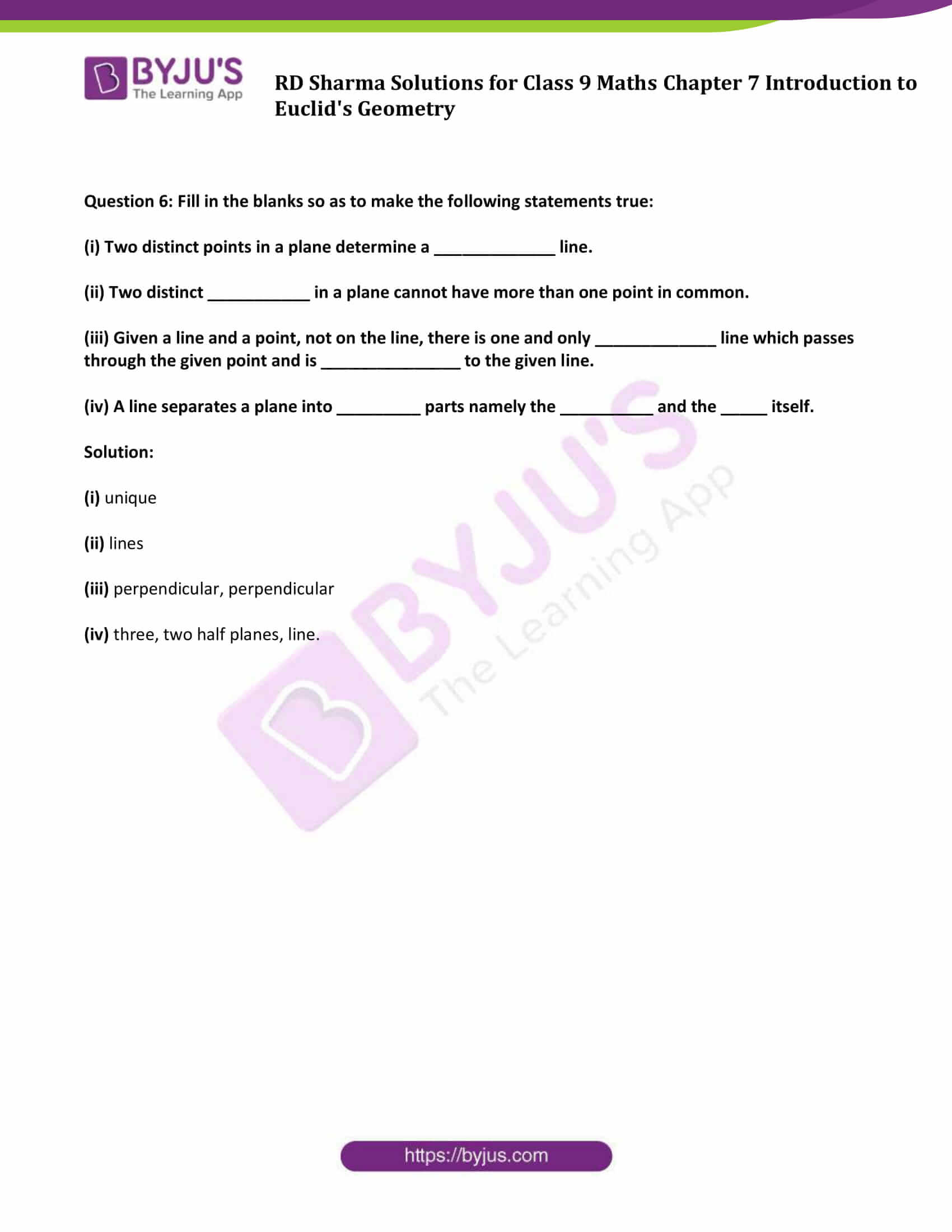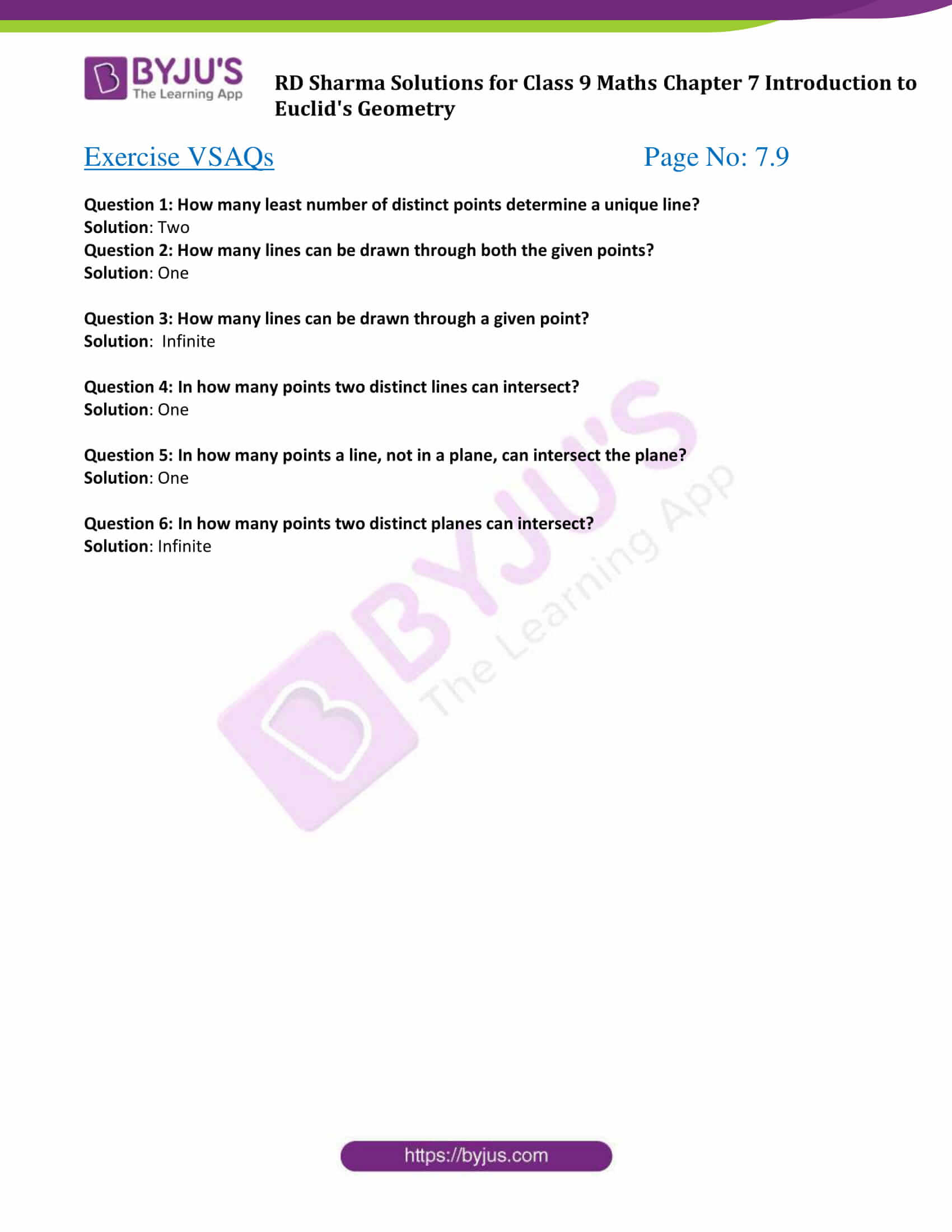### Exercise 7.1 Page No: 7.8

Question 1: Define the following terms.

(i) Line segment

(ii) Collinear points

(iii) Parallel lines

(iv) Intersecting lines

(v) Concurrent lines

(vi) Ray

(vii) Half-line

Solution:

(i) Line segment: The part of a line that connects two points or we can say that a shortest distance between the two points. A line segment is one-dimensional.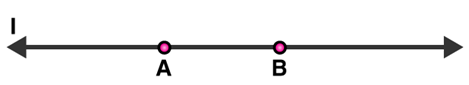Here AB is a line segment.

(ii) Collinear points: Two or more points are said to be collinear if all the points lie on same line.

(iii) Parallel lines : Two lines in a plane are said to be parallel lines if they do not intersect each other.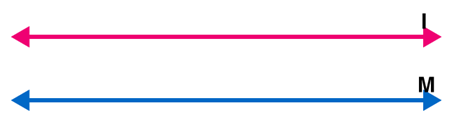Here l and m are parallel lines.

(iv) Intersecting lines: Two lines are intersecting if they have a common point. The common point is known as point of intersection.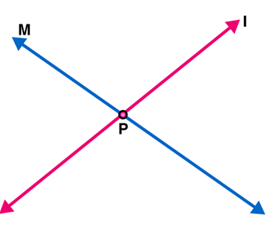Here l and M are intersecting lines. And P is point of intersection.

(v) Concurrent lines: Two or more lines are said to be concurrent if there is a point which lies on all of them.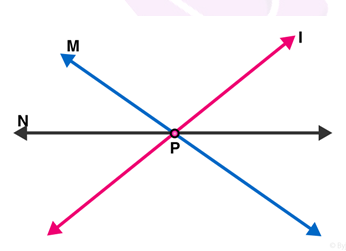Here l, m and n are concurrent lines.

(vi) Ray: A straight line extending from a point indefinitely in one direction only.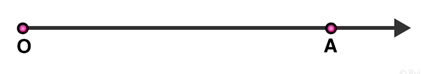Here OA is a ray.

(vii) Half-line: If A, B. C be the points on a line l, such that A lies between B and C, and we delete the point A from line l, the two parts of l that remain are each called a half-line.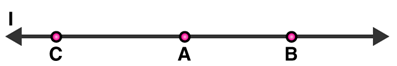Question 2:

(i) How many lines can pass through a given point?

(ii) In how many points can two distinct lines at the most intersect?

Solution:

(i) Infinitely many

(ii) One

Question 3:

(i) Given two points P and Q. Find how many line segments do they determine.

(ii) Name the line segments determined by the three collinear points P, Q and R.

Solution:

(i) One

(ii) PQ, QR, PR

Question 4: Write the truth value (T/F) of each of the following statements:

(i) Two lines intersect in a point.

(ii) Two lines may intersect in two points.

(iii) A segment has no length.

(iv) Two distinct points always determine a line.

(v) Every ray has a finite length.

(vi) A ray has one end-point only.

(vii) A segment has one end-point only.

(viii) The ray AB is same as ray BA.

(ix) Only a single line may pass through a given point.

(x) Two lines are coincident if they have only one point in common

Solution:

(i) False

(ii) False

(iii) False

(iv) True

(v) False

(vi) True

(vii) False

(viii) False

(ix) False

(x) False

Question 5: In the below figure, name the following:

(i) Five line segments

(ii) Five rays

(iii) Four collinear points

(iv) Two pairs of non–intersecting line segments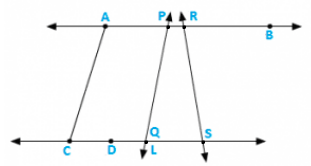Solution:

(i) Five line segments AB, CD, AC, PQ. DS

(ii) Five rays :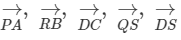(iii) Four collinear points. C, D, Q, S

(iv) Two pairs of non–intersecting line segments AB and CD, PB and LS.

Question 6: Fill in the blanks so as to make the following statements true:

(i) Two distinct points in a plane determine a _____________ line.

(ii) Two distinct ___________ in a plane cannot have more than one point in common.

(iii) Given a line and a point, not on the line, there is one and only _____________ line which passes through the given point and is _______________ to the given line.

(iv) A line separates a plane into _________ parts namely the __________ and the _____ itself.

Solution:

(i) unique

(ii) lines

(iii) perpendicular, perpendicular

(iv) three, two half planes, line.

### Exercise VSAQs Page No: 7.9

Question 1: How many least number of distinct points determine a unique line?

Solution: Two

Question 2: How many lines can be drawn through both the given points?

Solution: One

Question 3: How many lines can be drawn through a given point?

Solution: Infinite

Question 4: In how many points two distinct lines can intersect?

Solution: One

Question 5: In how many points a line, not in a plane, can intersect the plane?

Solution: One

Question 6: In how many points two distinct planes can intersect?

Solution: Infinite

### RD Sharma Solutions for Class 9 Maths Chapter 7 Introduction to Euclid’s Geometry

In the 7th Chapter of Class 9 RD Sharma Solutions students will study important concepts on Introduction to Euclid’s Geometry as listed below:

• Introduction to Euclid’s Geometry
• Postulates and Theorems
• Properties of point and lines
• Parallel Lines
• Intersecting Lines
• Line segment
• Interior point of a line segment
• Congruence of line segments
• Length axioms of a line segment
• Distance between two points

## Frequently Asked Questions on RD Sharma Solutions for Class 9 Maths Chapter 7

### How RD Sharma Solutions for Class 9 Maths Chapter 7 is helpful for exam preparation?

RD Sharma Solutions for Class 9 Maths Chapter 7 are created by the BYJU’S expert faculty to help students in the effective preparation of their examinations. These solutions created in a comprehensive manner help students to solve the problems comfortably and clear their doubts immediately. Students can make use of these solutions to prepare for their upcoming Board Exams by covering the whole syllabus, based on the RD Sharma guidelines.

### How many exercises are present in RD Sharma Solutions for Class 9 Maths Chapter 7?

There are 2 exercises present in RD Sharma Solutions for Class 9 Maths Chapter 7. The topics covered under these exercises are,
1. Introduction to Euclid’s Geometry
2. Postulates and Theorems
3. Properties of point and lines
4. Parallel Lines
5. Intersecting Lines
6. Line segment
7. Interior point of a line segment
8. Congruence of line segments
9. Length axioms of a line segment
10. Distance between two points

### What are the benefits of following RD Sharma Solutions for Class 9 Maths Chapter 7?

RD Sharma Solutions for Class 9 Maths Chapter 7 is useful for students as it helps them to score well in the class exams. We, in our aim to help students, have devised detailed chapter-wise solutions for them to understand the concepts easily. We follow the latest syllabus while creating the RD Sharma Solutions and it is framed in accordance with the exam pattern of the CBSE Board. These solutions are designed by subject matter experts who have assembled model questions covering all the exercise questions from the textbook.